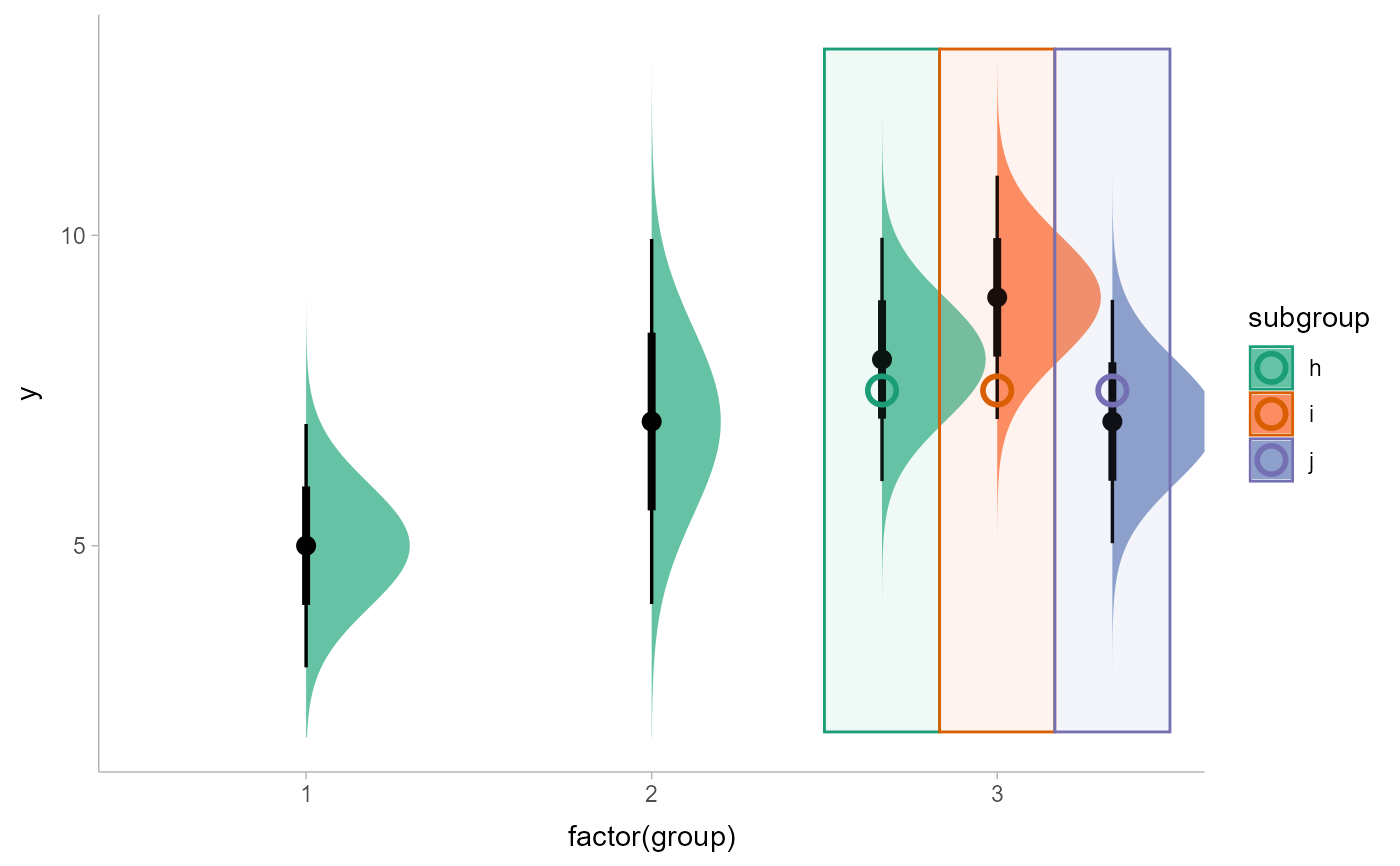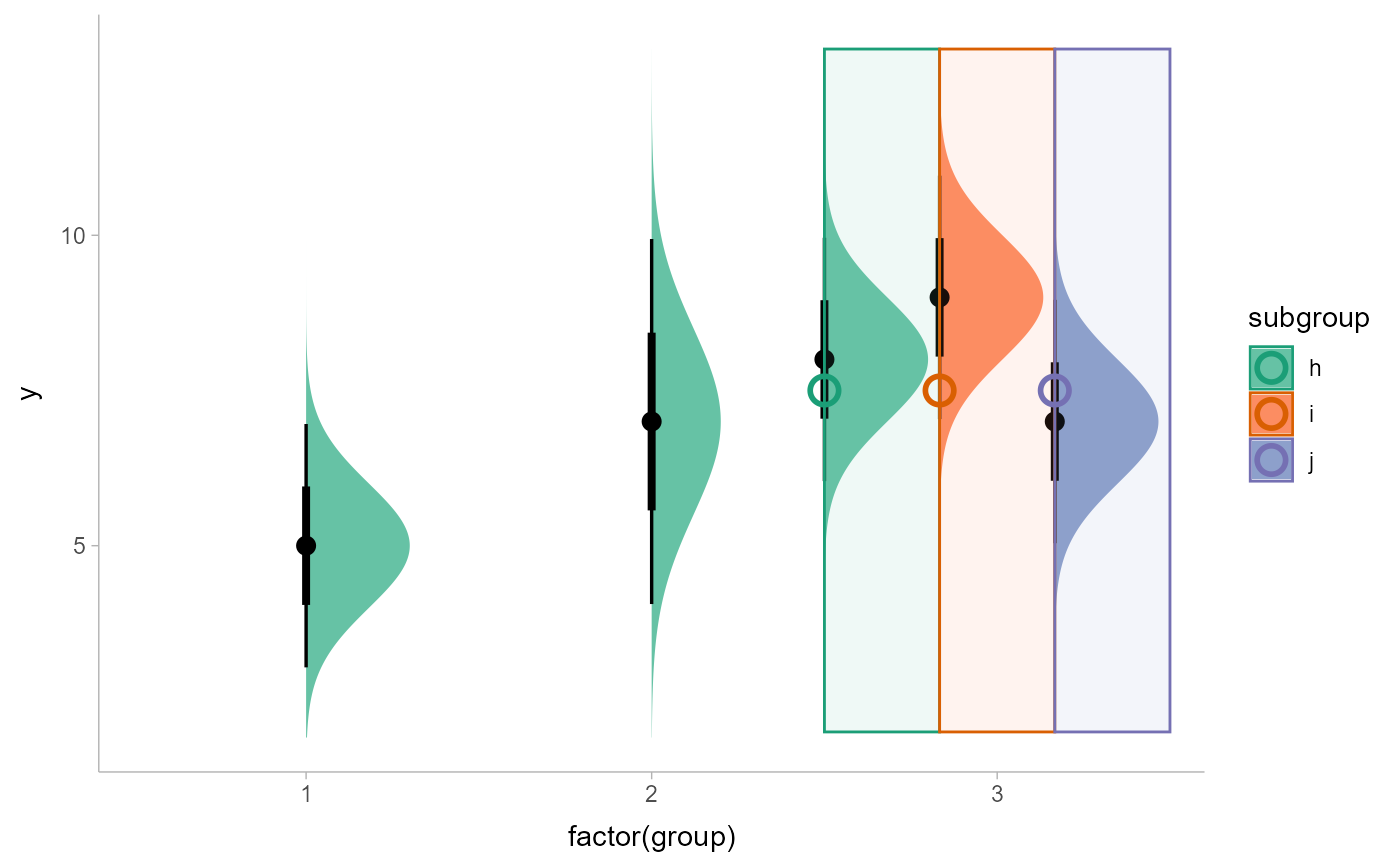A justification-preserving variant of ggplot2::position_dodge() which preserves the vertical position of a geom while adjusting the horizontal position (or vice versa when in a horizontal orientation). Unlike ggplot2::position_dodge(), position_dodgejust() attempts to preserve the "justification" of x positions relative to the bounds containing them (xmin/xmax) (or y positions relative to ymin/ymax when in a horizontal orientation). This makes it useful for dodging annotations to geoms and stats from the geom_slabinterval() family, which also preserve the justification of their intervals relative to their slabs when dodging.

position_dodgejust(
width = NULL,
preserve = c("total", "single"),
justification = NULL
)

## Arguments

width

Dodging width, when different to the width of the individual elements. This is useful when you want to align narrow geoms with wider geoms. See the examples.

preserve

Should dodging preserve the "total" width of all elements at a position, or the width of a "single" element?

justification

Justification of the point position (x/y) relative to its bounds (xmin/xmax or ymin/ymax), where 0 indicates bottom/left justification and 1 indicates top/right justification (depending on orientation). This is only used if xmin/xmax/ymin/ymax are not supplied; in that case, justification will be used along with width to determine the bounds of the object prior to dodging.

## Examples


library(dplyr)
library(ggplot2)
library(distributional)

dist_df = tribble(
~group, ~subgroup, ~mean, ~sd,
1,          "h",     5,   1,
2,          "h",     7,   1.5,
3,          "h",     8,   1,
3,          "i",     9,   1,
3,          "j",     7,   1
)

# An example with normal "dodge" positioning
# Notice how dodge points are placed in the center of their bounding boxes,
# which can cause slabs to be positioned outside their bounds.
dist_df %>%
ggplot(aes(
x = factor(group), ydist = dist_normal(mean, sd),
fill = subgroup
)) +
stat_halfeye(
position = "dodge"
) +
geom_rect(
aes(xmin = group, xmax = group + 1, ymin = 2, ymax = 13, color = subgroup),
position = "dodge",
data = . %>% filter(group == 3),
alpha = 0.1
) +
geom_point(
aes(x = group, y = 7.5, color = subgroup),
position = position_dodge(width = 1),
data = . %>% filter(group == 3),
shape = 1,
size = 4,
stroke = 1.5
) +
scale_fill_brewer(palette = "Set2") +
scale_color_brewer(palette = "Dark2")# This same example with "dodgejust" positioning. For the points we
# supply a justification parameter to position_dodgejust which mimics the
# justification parameter of stat_halfeye, ensuring that they are
# placed appropriately. On slabinterval family geoms, position_dodgejust()
# will automatically detect the appropriate justification.
dist_df %>%
ggplot(aes(
x = factor(group), ydist = dist_normal(mean, sd),
fill = subgroup
)) +
stat_halfeye(
position = "dodgejust"
) +
geom_rect(
aes(xmin = group, xmax = group + 1, ymin = 2, ymax = 13, color = subgroup),
position = "dodgejust",
data = . %>% filter(group == 3),
alpha = 0.1
) +
geom_point(
aes(x = group, y = 7.5, color = subgroup),
position = position_dodgejust(width = 1, justification = 0),
data = . %>% filter(group == 3),
shape = 1,
size = 4,
stroke = 1.5
) +
scale_fill_brewer(palette = "Set2") +
scale_color_brewer(palette = "Dark2")